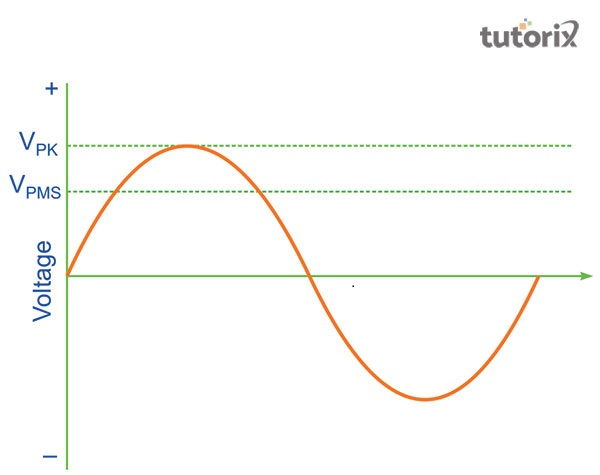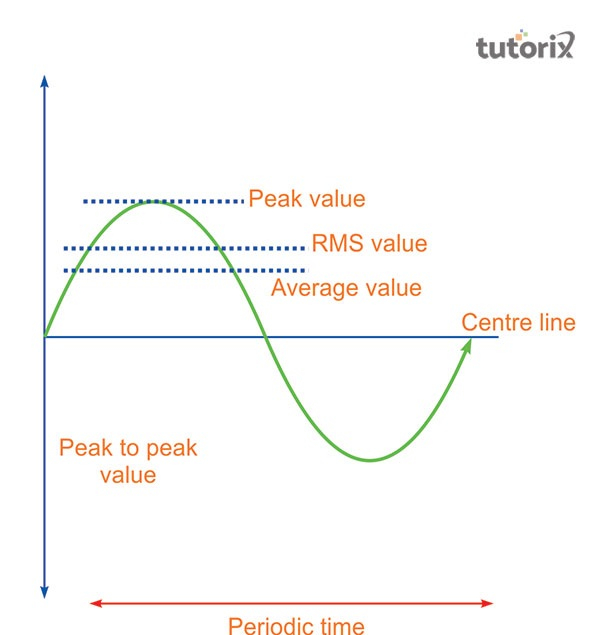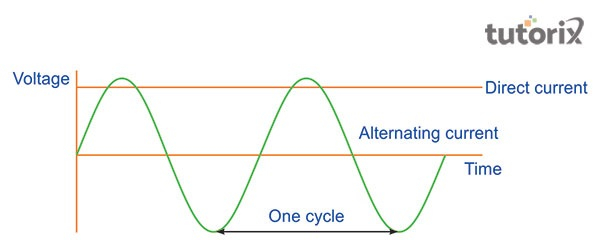# RMS Value Alternating Current

## Introduction

The root mean square of the current value is instantaneous. This value of the alternating current is denoted as a value of a stable current. this steady current helps in the generation of an equal amount of heat in a particular resistance within a given period as it is done by a.c current. This happens in the same manner when the ac is regulated across a similar resistance for the same period.

## What Is Alternating Current?

The value of an alternating current can be illustrated as a current flow that alters its magnitude and value of polarity at the regular time intervals. As opined by schavemaker & van der sluis (2017), this form of current can further be defined as the electrical current that changes continuously or it can reverse its direction. This aspect is totally opposite to that of direct current which forever flows in one particular direction.

The value of the alternating current (ac) , tends to attain its maximum value and then gradually drops back to zero after the completion of the positive cycle. The particles then change their direction and attain the maximum in an opposite direction after which the alternating current again changes to that value as it was originally, this change occurs after the completion of one negative state. This cycle continues to repeat itself.

These alternating currents are further accompanied by alternating voltages. Alternating current tends to easily transform from a voltage of high level to a voltage of lower level.Figure 1: Alternating Current

## RMS Value of Alternating Current

Rms is the short form of root mean square of instant current values. The root mean square value of these alternating currents is provided by a direct current that flows through a given resistance. Root mean square value of alternating current is always higher than the average value of the flowing current. As opined by mirković et al. (2018), the root mean square value in the case of sinusoidal wave current can be obtained by the area that is covered in a specific half-cycle. This value applies to all the forms of waves, be it Sinusoidal or Non-Sinusoidal, Symmetrical or Asymmetrical. This value is further noted using $\mathrm{I_{rms}}$ or $\mathrm{I_v}$.

The RMS Value of the alternating current is obtained from this formula

$\mathrm{I_{rms}\:=\:I_0/\sqrt{2}}$ which equals 0.707 $\mathrm{I_0}$.Figure 2: RMS Value of AC Current

## Alternating Current: Applications

The alternating current is associated with the flow of current which is mostly used in different apparatus. Some of the prime examples of the usage of alternating current include audio signals and radio signals, the alternating current has multiple advantages over direct current.

Alternating current is capable of travelling longer distances than direct current without additional loss of energy.Figure 3: AC and DC

The alternating current is mostly used in homes and offices because of its ease of travel in a larger expanse of area. It is to be noted that the alternating current is able to be converted to and from the high voltages with the use of transformers. The ac is also able to power electric motors which further helps in the conversion of electrical energy into mechanical energy. Due to these aspects, the working mechanism of refrigerators and electric dishwashers follows the principle of alternating current.

## Alternating current waveform

The most common waveform of alternating current in most of the circuits is sinusoidal, in this case, the positive half period corresponds to the positive direction of current flow and it is similar in the opposite scenario (europa 2022). a square or a triangular waveform can also be established that will help in representing the alternating current waveform.

The audio amplifiers deal with music signals or analogue voices that lead to the production of irregular ac waves.

## RMS value of AC value wave

The root mean square value is denoted as the square root of the means of squares of the values that are instantaneous. According to taheri & razavi (2018), this can further be described as a given amount of alternating current power that liberates the same heating effect as the dc power it is equivalent to.

## Conclusion

The alternating current is the most efficient form of a flow of current that is used in home and office areas because of its efficiency through an expanse without losing much energy. The root means square of the waves of this alternating current is noted by its instantaneous values. This value of the current is denoted by the direct current that flows through a particular resistance. In comparison to the average value, the root mean square value is always higher. This Rms value is applicable in all the forms of waves including sinusoidal, symmetrical, non-sinusoidal and asymmetrical waves.

## FAQs

Q1. What Is The Nature Of The Normal Waveforms Of Alternating Current?

Ans. Any Normal Waveform Of The Alternating Current As Found In Most Of The Circuits Is Generally Sinusoidal. In This Case, The Positive Half Period Of The Curve Corresponds To The Positive Direction Of The Current. On The Other Hand, The Positive Direction Corresponds With The Positive Half Period As Well.

Q2. How Can One Obtain The RMS Values Of Alternating Current?

Ans. The Rms value of the current is mostly obtained through pictorial representation. This value thus is generally obtained from the graphical method or analytical method of representation.

Q3. What Is The Use Of The RMS Value In An AC Source?

Ans. The RMS Value of the AC source determines its efficiency. The Root Mean Square value of a particular Alternating Current Source helps in the determination of its capability of Energy Transfer.

Q4. How Is AC More Helpful Than DC?

Ans. The alternating current devices are very durable since the power dissipation of the alternating current is lesser compared to the direct current. Henceforth, the transmission of electric power is more cost-efficient in the alternating current.